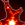# The Hipster Effect: An IPython Interactive Exploration¶

This notebook originally appeared as a post on the blog Pythonic Perambulations. The content is BSD licensed. It has been adapted to use HoloViews by Philipp Rudiger.

This week I started seeing references all over the internet to this paper: The Hipster Effect: When Anticonformists All Look The Same. It essentially describes a simple mathematical model which models conformity and non-conformity among a mutually interacting population, and finds some interesting results: namely, conformity among a population of self-conscious non-conformists is similar to a phase transition in a time-delayed thermodynamic system. In other words, with enough hipsters around responding to delayed fashion trends, a plethora of facial hair and fixed gear bikes is a natural result.

Also naturally, upon reading the paper I wanted to try to reproduce the work. The paper solves the problem analytically for a continuous system and shows the precise values of certain phase transitions within the long-term limit of the postulated system. Though such theoretical derivations are useful, I often find it more intuitive to simulate systems like this in a more approximate manner to gain hands-on understanding.

## Mathematically Modeling Hipsters¶

We'll start by defining the problem, and going through the notation suggested in the paper. We'll consider a group of $N$ people, and define the following quantities:

• $\epsilon_i$ : this value is either $+1$ or $-1$. $+1$ means person $i$ is a hipster, while $-1$ means they're a conformist.
• $s_i(t)$ : this is also either $+1$ or $-1$. This indicates person $i$'s choice of style at time $t$. For example, $+1$ might indicated a bushy beard, while $-1$ indicates clean-shaven.
• $J_{ij}$ : The influence matrix. This is a value greater than zero which indicates how much person $j$ influences person $i$.
• $\tau_{ij}$ : The delay matrix. This is an integer telling us the length of delay for the style of person $j$ to affect the style of person $i$.

The idea of the model is this: on any given day, person $i$ looks at the world around him or her, and sees some previous day's version of everyone else. This information is $s_j(t - \tau_{ij})$.

The amount that person $j$ influences person $i$ is given by the influence matrix, $J_{ij}$, and after putting all the information together, we see that person $i$'s mean impression of the world's style is

$$m_i(t) = \frac{1}{N} \sum_j J_{ij} \cdot s_j(t - \tau_{ij})$$

Given the problem setup, we can quickly check whether this impression matches their own current style:

• if $m_i(t) \cdot s_i(t) > 0$, then person $i$ matches those around them
• if $m_i(t) \cdot s_i(t) < 0$, then person $i$ looks different than those around them

A hipster who notices that their style matches that of the world around them will risk giving up all their hipster cred if they don't change quickly; a conformist will have the opposite reaction. Because $\epsilon_i$ = $+1$ for a hipster and $-1$ for a conformist, we can encode this observation in a single value which tells us what which way the person will lean that day:

$$x_i(t) = -\epsilon_i m_i(t) s_i(t)$$

Simple! If $x_i(t) > 0$, then person $i$ will more likely switch their style that day, and if $x_i(t) < 0$, person $i$ will more likely maintain the same style as the previous day. So we have a formula for how to update each person's style based on their preferences, their influences, and the world around them.

But the world is a noisy place. Each person might have other things going on that day, so instead of using this value directly, we can turn it in to a probabilistic statement. Consider the function

$$\phi(x;\beta) = \frac{1 + \tanh(\beta \cdot x)}{2}$$

We can plot this function quickly:

In :
import numpy as np
import holoviews as hv
hv.notebook_extension(bokeh=True, width=90)HoloViewsJS, BokehJS successfully loaded in this cell.